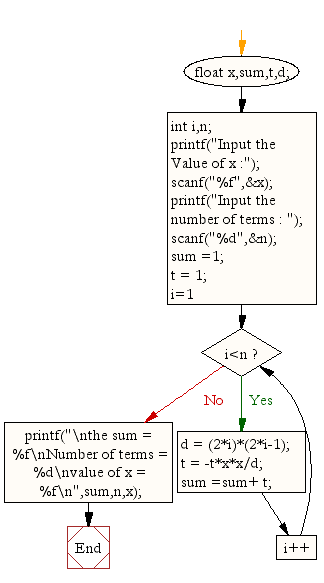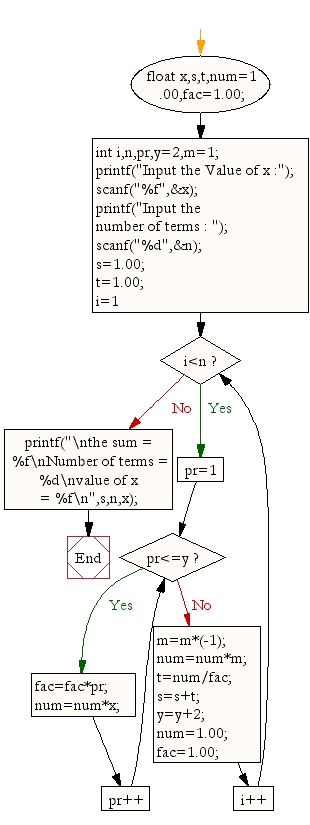﻿ C : Compute the sum of the series [ 1-X^2/2!+X^4/4!- ...]# C Exercises: Calculate the sum of the series [ 1-X^2/2!+X^4/4!- .........]

## C For Loop: Exercise-18 with Solution

Write a program in C to find the sum of the series [ 1-X^2/2!+X^4/4!- .........].

Sample Solution:

C Code:

``````#include <stdio.h>
void main()
{
float x,sum,t,d;
int i,n;
printf("Input the Value of x :");
scanf("%f",&x);
printf("Input the number of terms : ");
scanf("%d",&n);
sum =1; t = 1;
for (i=1;i<n;i++)
{
d = (2*i)*(2*i-1);
t = -t*x*x/d;
sum =sum+ t;
}
printf("\nthe sum = %f\nNumber of terms = %d\nvalue of x = %f\n",sum,n,x);
}
```
```

Flowchart:or

``````#include <stdio.h>

void main()
{
float x,s,t,num=1.00,fac=1.00;
int i,n,pr,y=2,m=1;

printf("Input the Value of x :");
scanf("%f",&x);
printf("Input the number of terms : ");
scanf("%d",&n);
s=1.00; t=1.00;

for (i=1;i<n;i++)
{
for(pr=1;pr<=y;pr++)
{
fac=fac*pr;
num=num*x;

}
m=m*(-1);
num=num*m;
t=num/fac;
s=s+t;
y=y+2;
num=1.00;
fac=1.00;

}
printf("\nthe sum = %f\nNumber of terms = %d\nvalue of x = %f\n",s,n,x);
}
```
```

Sample Output:

```Input the Value of x :2
Input the number of terms : 5

the sum = -0.415873
Number of terms = 5
value of x = 2.000000
```

Flowchart:C Programming Code Editor:

Improve this sample solution and post your code through Disqus.

What is the difficulty level of this exercise?

Test your Programming skills with w3resource's quiz.

﻿